Scattering and tunnelling

Start this free course now. Just create an account and sign in. Enrol and complete the course for a free statement of participation or digital badge if available.

Free course

# Conclusion

Session 1

Scattering is a process in which incident particles interact with a target and are changed in nature, number, speed or direction of motion as a result. Tunnelling is a quantum phenomenon in which particles that are incident on a classically impenetrable barrier are able to pass through the barrier and emerge on the far side of it.

Session 2

In one dimension, wave packets scattered by finite square barriers or wells generally split into transmitted and reflected parts, indicating that there are non-zero probabilities of both reflection and transmission. These probabilities are represented by the reflection and transmission coefficients R and T. The values of R and T generally depend on the nature of the target and the properties of the incident particles. If there is no absorption, creation or destruction of particles, R + T = 1.

Session 3

Unnormalisable stationary-state solutions of Schrödinger's equation can be interpreted in terms of steady beams of particles. A term such as Aei(kx − ωt) can be associated with a beam of linear number density n = |A|2 travelling with speed v =k/m in the direction of increasing x. Such a beam has intensity j = nv. In this approach, T = jtrans/jinc and R = jref/jinc.

For particles of energy E0 > V0, incident on a finite square step of height V0, the transmission coefficient is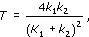where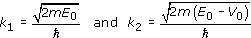are the wave numbers of the incident and transmitted beams. For a finite square well or barrier of width L, the transmission coefficient can be expressed as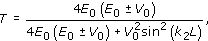where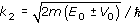, with the plus signs being used for a well and the minus signs for a barrier. Transmission resonances, at which T = 1 and the transmission is certain, occur when k2L = Nwhere N is an integer.

Travelling wave packets and steady beams of particles can both be thought of as representing flows of probability. In one dimension such a flow is described by the probability current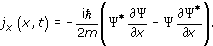In three dimensions, scattering is described by the total cross-section, σ, which is the rate at which scattered particles emerge from the target per unit time per unit incident flux. For any chosen direction, the differential cross-section tells us the rate of scattering into a small cone of angles around that direction. At very high energies, total cross-sections are dominated by inelastic effects due to the creation of new particles.

Session 4

Wave packets with a narrow range of energies centred on E0 can tunnel though a finite square barrier of height V0 > E0. In a stationary-state approach, solutions of the time-independent Schrödinger equation in the classically forbidden region contain exponentially growing and decaying terms of the form Ce−αx and Deαx, where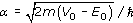is the attenuation coefficient. The transmission coefficient for tunnelling through a finite square barrier of width L and height V0 is approximately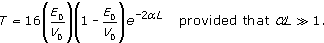Such a transmission probability is small and decreases rapidly as the barrier width L increases.

Session 5

Square barriers and wells are poor representations of the potential energy functions found in Nature. However, if the potential V(x) varies smoothly as a function of x the transmission coefficient for tunnelling of energy E0can be roughly represented by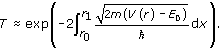This approximation can be used to provide a successful theory of nuclear alpha decay as a tunnelling phenomenon. It can also account for the occurrence of nuclear fusion in stellar cores, despite the relatively low temperatures there. In addition, it explains the operation of the scanning tunnelling microscope which can map surfaces on the atomic scale.

Glossary and Physics Toolkit

Attached below are PDFs of the original glossary and the Physics Toolkit, you may find it useful to refer to these documents as you work through the course.

Click to view glossary [Tip: hold Ctrl and click a link to open it in a new tab. (Hide tip)] . (16 pages, 0.4 MB)

Click to view toolkit. (17 pages, 0.4 MB)

SM358_1